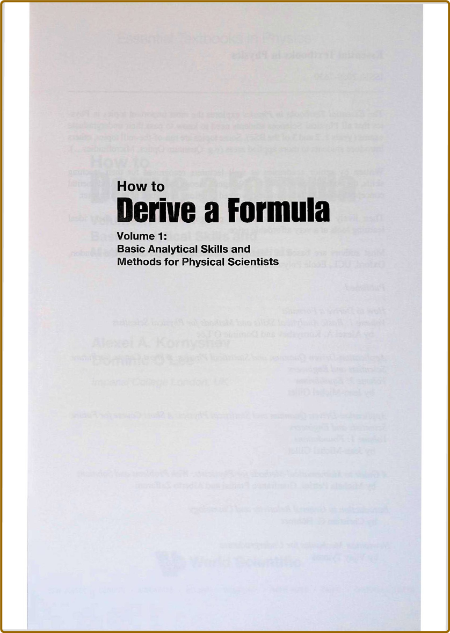# /# Kornyshev A How to derive a formula Vol 1 2020pdf | 226.97 MB | English | Isbn:‎ 1786346346 | Author: Alexei A Kornyshev | Year: 2020

Description:

Will artificial intelligence solve all problems, making scientific formulae redundant? The authors of this book would argue that there is still a vital role in formulating them to make sense of the laws of nature. To derive a formula one needs to follow a series of steps; last of all, check that the result is correct, primarily through the analysis of limiting cases. The book is about unravelling this machinery.Mathematics is the 'queen of all sciences', but students encounter many obstacles in learning the subject — familiarization with the proofs of hundreds of theorems, mysterious symbols, and technical routines for which the usefulness is not obvious upfront. Those interested in the physical sciences could lose motivation, not seeing the wood for the trees.How to Derive a Formula is an attempt to engage these learners, presenting mathematical methods in simple terms, with more of an emphasis on skills as opposed to technical knowledge. Based on intuition and common sense rather than mathematical rigor, it teaches students from scratch using pertinent examples, many taken across the physical sciences.This book provides an interesting new perspective of what a mathematics textbook could be, including historical facts and humour to complement the material.

Category:Differential Equations, Mathematical Physics, CalculusIf You Got Any Problem With Links ... Just PM US (Click Here) or